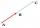# Unit vector 2D

Determine coordinates of unit vector to vector AB if A[-6; 8], B[-18; 10].

Result

x =  -0.99
y =  0.16

#### Solution:Leave us a comment of example and its solution (i.e. if it is still somewhat unclear...):

Showing 1 comment:Tza0987
097=134
134=824
824=650
650=?#### To solve this verbal math problem are needed these knowledge from mathematics:

For Basic calculations in analytic geometry is helpful line slope calculator. From coordinates of two points in the plane it calculate slope, normal and parametric line equation(s), slope, directional angle, direction vector, the length of segment, intersections the coordinate axes etc. Two vectors given by its magnitudes and by included angle can be added by our vector sum calculator. Pythagorean theorem is the base for the right triangle calculator.

## Next similar examples:

1. TriangleTriangle KLM is given by plane coordinates of vertices: K[14, -2] L[8, 13] M[-1, -18]. Calculate its area and itsinterior angles.
2. CrossroadsThe rectangular crossroads comes passenger car and an ambulance, the ambulance left. Passenger car is at 43 km/h and ambulance 52 km/h. Calculate such a relative speed of the ambulance moves to the car.
3. Airplane navigationAn airplane leaves an airport and flies due west 120 miles and then 150 miles in the direction S 44.1°W. How far is the plane from the airport (round to the nearest mile)?
4. VectorCalculate length of the vector v⃗ = (9.75, 6.75, -6.5, -3.75, 2).
5. Two forcesTwo forces with magnitudes of 25 and 30 pounds act on an object at angles of 10° and 100° respectively. Find the direction and magnitude of the resultant force. Round to two decimal places in all intermediate steps and in your final answer.
6. Angle between vectorsFind the angle between the given vectors to the nearest tenth of a degree. u = (-22, 11) and v = (16, 20)
7. Rectangle 39Find the perimeter and area of the rectangular with vertices (-1, 4), (0,4), (0, -1), and (-4, 4)
8. Linear independenceDetermine if vectors u=(-4; -5) and v=(20; 25) are linear Linear dependent.
9. VectorsVector a has coordinates (8; 10) and vector b has coordinates (0; 17). If the vector c = b - a, what is the magnitude of the vector c?
10. 3d vector componentThe vector u = (3.9, u3) and the length of the vector u is 12. What is is u3?
11. Find the 5Find the equation with center at (1,20) which touches the line 8x+5y-19=0
12. LineLine p passing through A[-10, 6] and has direction vector v=(3, 2). Is point B[7, 30] on the line p?
13. CylindersArea of the side of two cylinders is same rectangle of 50 cm × 11 cm. Which cylinder has a larger volume and by how much?
14. Equilateral cylinderEquilateral cylinder (height = base diameter; h = 2r) has a volume of V = 199 cm3 . Calculate the surface area of the cylinder.
15. Speed of Slovakian trainsRudolf decided to take the train from the station 'Trnava' to 'Zemianske Kostoľany'. In the train timetables found train R 725 Remata : km0Bratislava hl.st.12:574Bratislava-Vinohrady13:0113:0219Pezinok13:1213:1346Trnava13:3013:3263Leopoldov13:4514:0168H
16. SegmentsWhich of the pairs of numbers on the number line encloses the longest segment: ?
17. CircleCircle is given by centre on S[-7; 10] and maximum chord 13 long. How many intersect points have circle with the coordinate axes?